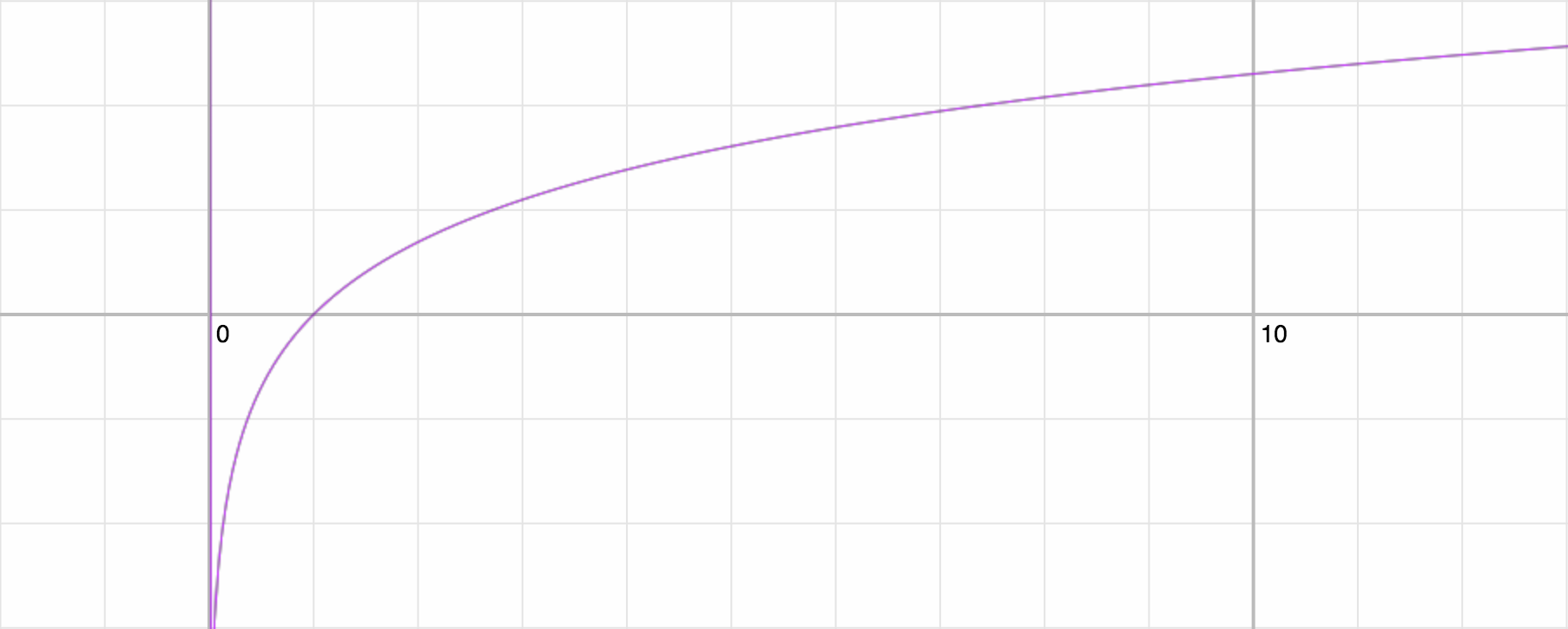I've read many articles online with differing, vague, descriptions of entropy. But I recently found out that it has a clear mathematical representation!

Suppose you're performing an experiment which has 5 possible outcomes. We'll label them $$i = {1, ..., 5}$$. And suppose that they each occur with probability $$p(i)$$. $$p$$ is called a distribution, which is just a fancy name for a function that gives you probabilities.

You can associate a value with the distribution $$p$$ called entropy. It tells you how many of the outcomes are "reasonably likely". If only one outcome is likely, the entropy is low. If many are likely, it's high.

I'm going to show you the formula for entropy and then try to make sense of it.

Here it is... $$E(p) = - \sum_{i=1}^{i=N} p(i) \log p(i)$$

Let's pick this apart. There's a sum over all possible outcomes. For each term in the sum, you multiply the probability of the outcome with the log of the probability. And there's a negative sign out in front.

In case you've forgotten what a log graph looks like, here it is...Here's an important detail: probabilities are always between $$0$$ and $$1$$. And from the graph above, we can see that $$\log(x)$$ is negative when $$x < 1$$. So $$\log p(i)$$ is always negative.

This explains why there's a minus sign in our formula for entropy. Each term in the sum is negative, so the result of the sum negative. It feels good to have entropy be a positive number, so we stick a minus sign in front.

Next, let's find the entropy for some simple distributions.

Suppose you have an experiment with $$N$$ outcomes. And let's say that one outcome has probability $$1$$ and all other outcomes have probability $$0$$. What's the entropy of this distribution?

Consider the outcome with probability $$1$$. It's term in the sum is $$1 \log 1$$, which is zero because $$\log 1$$ is zero. And all the other terms are zero because $$p(i)$$ is zero. So the entropy is $$0$$.

Okay, let's consider another distribution. Suppose all outcomes have equal probability. Then $$p(i) = \dfrac{1}{N}$$ for each outcome. The sum becomes...

\begin{aligned} E(p) & = - \sum_{i=1}^{i=N}\dfrac{1}{N} \log \dfrac{1}{N} \\ & = - \log \dfrac{1}{N} \\ & = \log N \end{aligned}

In words: when all $$N$$ outcomes have equal probability, the entropy is $$\log N$$. This is the maximum possible entropy for a set of $$N$$ outcomes.

These examples match what I said earlier! When entropy is high, many outcomes are likely. When the entropy is low, very few outcomes are likely. In the first example only one outcome is likely, so the entropy is low. In the second example, all outcomes are equally likely, so the entropy is high.

The demo below lets you play with a probability distribution with $$5$$ outcomes and see its entropy. You can drag the gray handles to move a bar up or down.

Try making all the bars equal. You should see that the entropy is $$\log 5$$ (or roughly $$2.32$$), as per the formula above.

Something tricky to be aware of: the sum of probabilities of all outcomes should equal $$1$$. When you move a bar around, the demo will shift the probabilities of other bars so that they all sum to $$1$$.

That's all for now! I'd like to talk about the applications of entropy, but I don't know enough about that yet. When I learn more, I'll write a part 2.